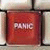## If Statement isn’t evaluating out correctlyTags:
IF statement
VB.NET
VB.NET 2005
VB.NET 2005 Express Edition
VB.NET Express Edition
I am trying to learn VB.Net using VB Studio 2005 Express edition and a pretty good text book. I thought I was beginning to understand concepts until this exercise came up - now I wonder... No matter what value I put into txtOrderTotal.Text, I get the same dDiscountTotal... This is 'hand-fed' code that is directly entered from the textbook (and I verified accuracy). Is it maybe something to do with the text-to-decimal change? Here is the code: Dim dOrderTotal As Decimal Dim dDiscountPct As Decimal Dim dDiscountAmount As Decimal Dim dInvoiceTotal As Decimal dOrderTotal = txtOrderTotal.Text If dOrderTotal >= 500 Then dDiscountPct = 0.2 ElseIf dOrderTotal >= 250 And dOrderTotal < 500 Then dDiscountPct = 0.15 ElseIf dOrderTotal >= 100 And dOrderTotal < 250 Then dDiscountPct = 0.1 Else dDiscountPct = 0 End If dDiscountAmount = dOrderTotal * dDiscountPct dInvoiceTotal = dOrderTotal - dDiscountAmount lblDiscountAmount.Text = dDiscountAmount lblInvoiceTotal.Text = dInvoiceTotal txtOrderTotal.Focus() Thanks in advance for any assistance, R Brown

Thanks. We'll let you know when a new response is added.

Guys,
I figured it out… the conditions weren’t being defined well enough for the ‘between’ values. Here are the changes I made to the If…Then… Else statements:

If dOrderTotal >= 500 Then
dDiscountPct = 0.2
ElseIf dOrderTotal >= 250 And dOrderTotal < 500 Then
dDiscountPct = 0.15
ElseIf dOrderTotal >= 100 And dOrderTotal < 250 Then
dDiscountPct = 0.1
Else ‘(dDiscountPct < 100)
dDiscountPct = 0
End If

I appreciate all of your assistance – you guys got me to thinking a little more clearly.

Ruth

## Discuss This Question: 6 Replies

Thanks. We'll let you know when a new response is added.
•And, what value are you getting for dDiscountTotal ?
report
•When you say "No matter what value I put into txtOrderTotal.Text, I get the same dDiscountTotal", you mean that you are entering values in the txtOrderTotal box at runtime, right ? Or are you trying assigning values to it programmatically ? I have just created a test project with a text box, two labels and a button, and copied your code, and it works ok for me.
report
•The code does look like it works. Do you mean lblDiscountAmount.Text = dDiscountAmount or lblInvoiceTotal.Text doesn't change?
report
• I will try to give a little better answer about what I am seeing... When I go into Runtime, no matter what value I enter for txtOrderTotal.Text, I get the same dDiscountTotal. If I put in 550 for txtOrderTotal.Text, I should see a 20% discount. If I put in 300, I should get a 15% discount. If I put in 100, I should see a 10% discount. Less than 100 should get no discount. What I am seeing is that for any OrderTotal (500, 300, 100, 50) I get the same 20% discount. It's like the code is ignoring the additional statements. Just reading through the code, it looks like it should work, but I am working on my first true exercise in this textbook and am probably overlooking something. That's why I asked about the use of the .text ending on the txtOrderTotal.text - could it not be converting the text to number correctly? I even tried it using the Select Case method & got the same thing... I really appreciate you guys trying to help me out. Ruth
report
•I'm glad you figured it out, and thanks for sharing, but I don't see the difference with the original code.
report
• The only difference in the code is that I added a 'between' value. Before, it only had > or >= values. I added the < value for the in between numbers that need to be >= one and < the other. I guess what made me think to try it was the fact that I have to do that when I code VBA in Access... Ruth
report